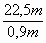# Translations of Encyclopedia about Mathematics

## Equations, Equations with Variables, Inequalities

Equations are mathematical statements in which there are two terms and expressions joined together by an equal sign or not equal sign.

Equations without Variables

An equation without a variable (unknown) is a mathematical statement which can be true (T) or false (F).

Example:

7 + 4 = 11 (T)
3 . 9 = 30 (F)

Equations with Variable (Unknowns)
We use equations with unknowns in our daily lives:

If for example we would like to wall-paper a room which has a circumference of 22.5m and we have wall-paper of width 90cm (=0.9m), we ask how much wall-paper we would need to buy to completely cover the room.

In this case, we would label the number of necessary rolls as x, which is an unknown. We know the width of the roll and we also know our value of 22.5m.

This gives us the equation:

0,9 m . x = 22,5 m

We find therefore that this problem is not difficult at all.

As you can see, we must first determine the quantity of all unknown values, which we can label as Mz. The quantity of rational numbers are by rule labelled as Q and real numbers as R.

The definition Md was first brought up in our previous subject of divisions. The definition is the set of all values which are unknown but restricted in terms of the task at hand. In our case, the definition was all real numbers.

Then we determine the set of possible solutions, Mv. This is the set of all values in which equality and inequality are met, or in which the statement is true.

By resolving an equation, we attain an application of rules of converting and transforming terms. In one or more steps, we try to isolate the unknown and carry it over to the opposite side of the equation from where all the known values are placed.

It is necessary to make sure that the equation truly applies and that one side equals the other. In other words, what occurs on one side of an equation must also occur on the other:

0,9 m · x = 22,5 m | : 0,9 m

x =x = 25

From this, it ensues that: Mv = { 25}

It is recommended to define a modification on one side which will also be applied to the other side of the equation (to keep the statement equal).

A major difference between equalities and inequalities is that expressions are joined together by such inequalities as greater than ( > ), less than ( < ), greater than or equal to ( ³ ), or less than or equal to ( £ ).

With inequalities without variables, we have statements which also may be true (T) or false (F), such as:

7 < 11 (T) 71 > 99 (F)

Resolving inequalities with variables is accomplished in the same manner as when resolving equations.

36 . x £ 90 / :36
x £ 90/36
x £ 2,5

If the variable x will be less than or equal to 2.5, the condition will have been met (the inequality is true) and we write the solution as Mv = { x Î R / x £ 2,5 } . In other words, the solution is the set of real numbers less than or equal to 2.5.

Translating Russian Ukrainian Translations Ukrainian Russian Translating Persian Arab Translations Arab Persian Translating TurkishEnter your search terms Submit search form

Copyright © KENAX, by Karel Kosman - All Rights Reserved Worldwide.